## LetsPlayMaths.Com

WELCOME TO THE WORLD OF MATHEMATICS

# Class 6 Angles

Angles

Acute Angle

Right Angle

Obtuse angle

Straight Angle

Reflex Angle

Zero Angle

Complete Angle

Protractor

Measurement of Angles Using Protractor

Congruent Angles

Angles Test

Angles Worksheet

## Angles

When two rays start from the same point as shown in the below figure is known as an angle.The common initial point 'O' is known as vertex. OA and OB are called sides of the angle. The symbol used to denote an angle is '∠'. So, we can name the above angle as ∠AOB or ∠BOA. There are various types of angles, they are mentioned below.

1. Acute angle
2. Right angle
3. Obtuse angle
4. Straight angle
5. Reflex angle
6. Zero angle
7. Complete angle

## Acute Angle

An angle which is more than 0° and less than 90° is known as acute angle.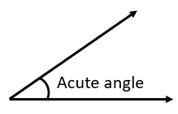## Right Angle

An angle whose measure is 90° is known as right angle.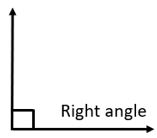## Obtuse angle

An angle which is more than 90° and less than 180° is known as obtuse angle.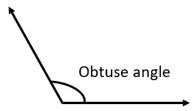## Straight Angle

An angle which is equal to 180° is known as straight angle.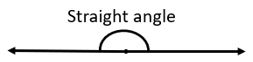## Reflex Angle

An angle whose measure lies between 180° and less than 360° is known as reflex angle.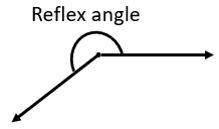## Zero Angle

An angle which is equal to 0° is known as zero angle.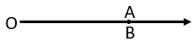Here ∠AOB is a zero angle.

## Complete Angle

An angle whose measure is 360° is known as complete angle.Here ∠AOB is a complete angle.

## Protractor

Protractor is a semi circular instrument by which we can measure angles. The mid point of its horizontal edge is known as central point. The stright angle at the central point is divided into 180 equal divisions, each measuring 1°. As we can see a protractor has two scales. The inner scale is anticlockwise bigins with 0° on the right and run upto 180° on the left. The outer clockwise scale begins 0° on the left and runs up to 180° on the right.## Measurement of Angles Using Protractor

Let's find out the measurement of below given angle ∠AOB.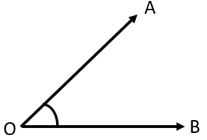Now place the protractor in such a way that it's centre is exactly on the vertex 'O' and base line lies on the side OB of the angle as shown in the below figure.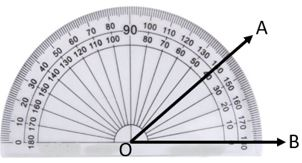Read off the mark through which the side OA passes starting from 0° on the right. We can see OA passes through 40° mark.
So, ∠AOB = 40°

Let's find out the measurment of below given angle ∠ZYZ.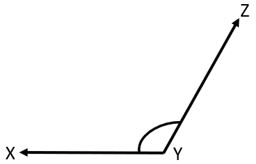Now place the protractor in such a way that it's centre is exactly on the vertex 'Y' and base line lies on the side XY of the angle as shown in the below figure.Read off the mark through which the side YZ passes starting from 0° on the left. We can see YZ passes through 120° mark.
So, ∠AOB = 120°

## Congruent Angles

Two angles having the same measure are known as congruent angles. In the below given figures both ∠PQR and ∠XYZ having 30°.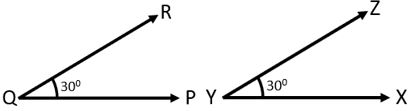Angle Test - 1

Angle Test - 2

## Class-6 Angle Worksheet

Angle Worksheet - 1

Angle Worksheet - 2